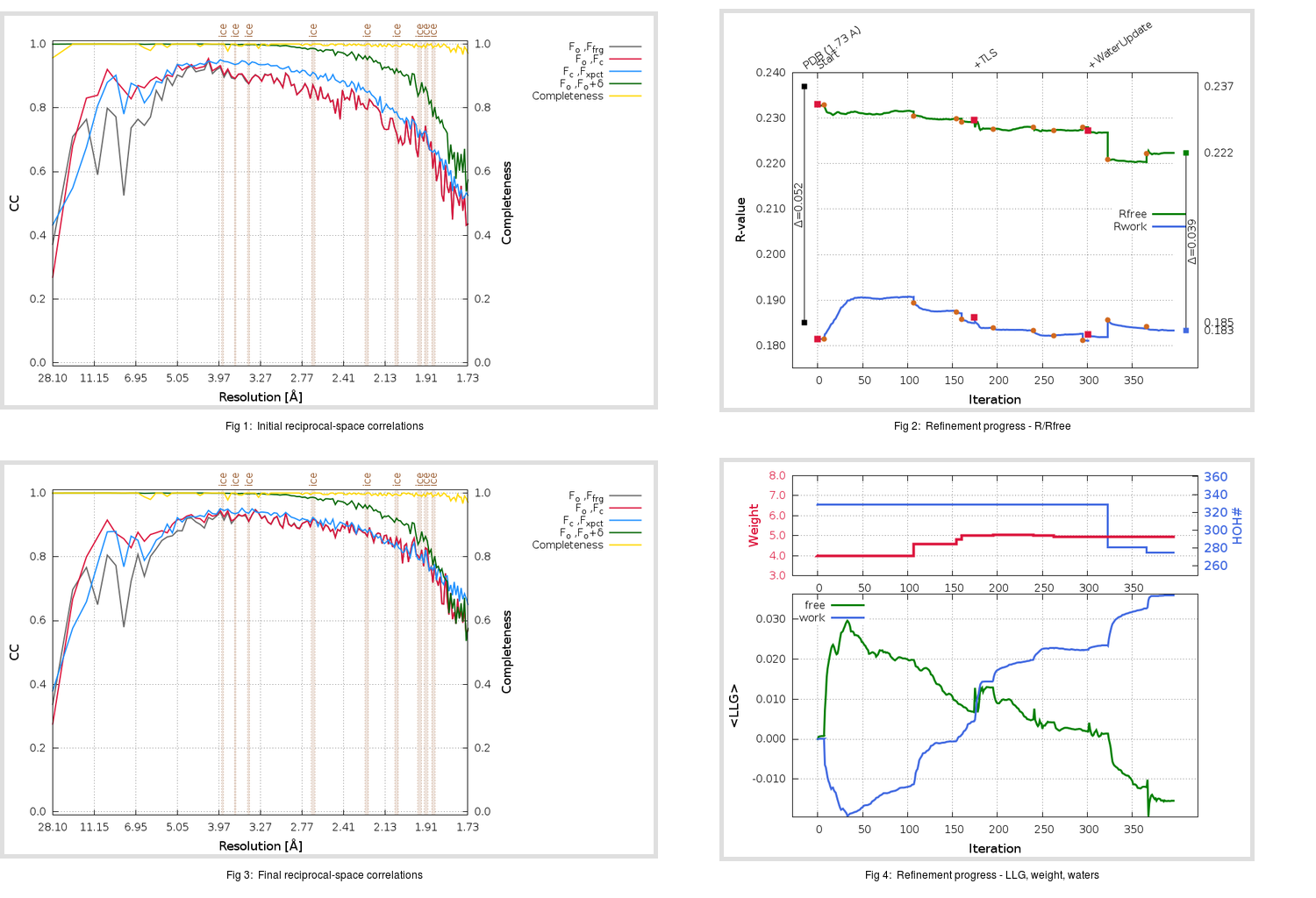Content:

```    Diffraction limits & principal axes of ellipsoid fitted to diffraction cut-off surface:
1.838         0.9954   0.0000  -0.0954       0.992 a* - 0.124 c*
1.747         0.0000   1.0000   0.0000       b*
1.651         0.0954   0.0000   0.9954       0.247 a* + 0.969 c*
```

## Deposited

` `
 Date deposited Date data collection Resolution R, Rfree 20200315 20200227 1.73 0.1830 0.2370

Molprobity (CCP4 7.0 version) summary:

```Ramachandran outliers =   0.33 %
favored =  97.35 %
Rotamer outliers      =   1.52 %
C-beta deviations     =     0
Clashscore            =   5.06
RMS(bonds)            =   0.0134
RMS(angles)           =   1.81
MolProbity score      =   1.53
Resolution            =   1.73
R-work                =   0.1830
R-free                =   0.2370
```

```Number of waters      =   329

<B> (all atoms) =   29.49 ( sd =   10.76 ) for       2733 non-hydrogen atoms
<B>   (protein) =   27.93 ( sd =    9.79 ) for       2370 non-hydrogen atoms
<B>     (water) =   40.37 ( sd =   10.63 ) for        329 non-hydrogen atoms
<B>    (others) =   32.72 ( sd =   15.71 ) for         34 non-hydrogen atoms

B min/max       (all non-hydrogen atoms) =   15.81 /   86.01
B min/max   (protein non-hydrogen atoms) =   15.81 /   78.41
B min/max     (water non-hydrogen atoms) =   16.09 /   86.01
B min/max     (other non-hydrogen atoms) =   18.94 /   53.77
```

## BUSTER (re-)refinement

` `

Molprobity (CCP4 7.0 version) summary:

```Ramachandran outliers =   0.33 %
favored =  98.34 %
Rotamer outliers      =   0.76 %
C-beta deviations     =     0
Clashscore            =   2.74
RMS(bonds)            =   0.0115
RMS(angles)           =   1.56
MolProbity score      =   1.06
Resolution            =   1.73
R-work                =   0.1834
R-free                =   0.2223
```

```Number of waters      =   275

<B> (all atoms) =   29.41 ( sd =    9.28 ) for       2679 non-hydrogen atoms
<B>   (protein) =   27.95 ( sd =    7.93 ) for       2370 non-hydrogen atoms
<B>     (water) =   41.27 ( sd =   10.55 ) for        275 non-hydrogen atoms
<B>    (others) =   35.46 ( sd =   16.98 ) for         34 non-hydrogen atoms

B min/max       (all non-hydrogen atoms) =   16.45 /   90.78
B min/max   (protein non-hydrogen atoms) =   16.45 /   69.11
B min/max     (water non-hydrogen atoms) =   17.54 /   90.78
B min/max     (other non-hydrogen atoms) =   23.08 /   58.03
```

Refinement progression:Results:

` `
 File Remark 5REC_aB_refine.01_03_refine.pdb.gz exact refinement commands are in header 5REC_aB_refine.01_03_refine.mtz.gz including original deposited data and several re-refinement map coefficients 5REC_aB_refine.01_03_BUSTER_model.cif.gz including any non-standard compound restraints 5REC_aB_refine.01_03_BUSTER_refln.cif.gz